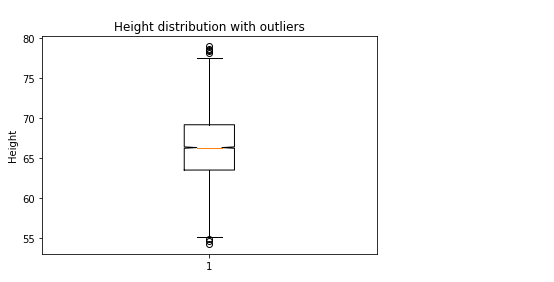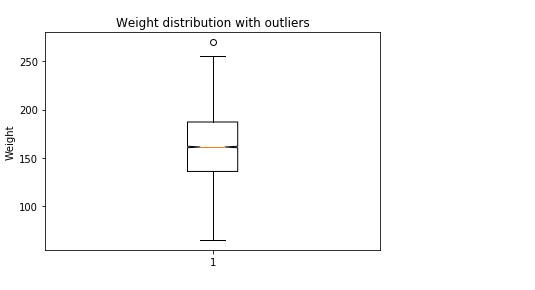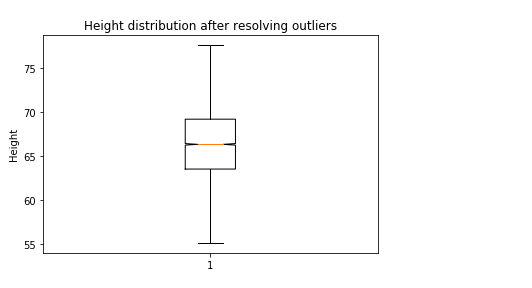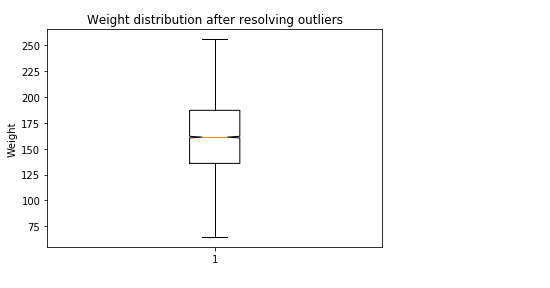• #5, First Floor, 4th Street Dr. Subbarayan Nagar Kodambakkam, Chennai-600 024 Landmark : Samiyar Madam
• pro@slogix.in
• +91- 81240 01111

### How to create simple linear regression model for the data set height and weight of male and female in python?

###### Description

To create a simple linear regression model for the given data set and analyse the summary and goodness of the model

###### Process

Find and resolve the Missing values

Find and resolve the Outliers

Split the data set for training and testing with ratio 80:20 so that training and testing data has 80% and 20% of the original data set respectively

Build the model

Fit the model using the training data

Test the model using the test data

Take the summary and analyse it

Building a simple linear regression model :

Simple linear regression model can be created in python in two different methods.They are

Using sklearn library

Using statsmodel api

Using sklearn library : Required libraries :

from sklearn.model_selection import train_test_split

from sklearn.linear_model import LinearRegression

from sklearn import metrics

Functions used :

To split the data - x_train, x_test, y_train, y_test = train_test_split(x, y, test_size=0.20, random_state=42)

To build the model - reg=LinearRegression()

To train the model - reg.fit(x_train_data,y_train_data)

To test the model - reg.predict(x_test_data)

To find the R -Squared value - reg.score(x_test,y_test)

To find the R-Squared value using metrics library - metrics.r2_score(y_test,y_pred)

Using statsmodel api : Required libraries :

import statsmodels.api as sm

Functions used :

To Train the model - model = sm.OLS(y_train,x_train)

To fit the model - results = model.fit()

To test the model - results.predict(x_test)

To take the summary -results.summary()

###### Sample Code

#import necessary libraries
import pandas as pd
import numpy as np
import matplotlib.pyplot as plt
from sklearn.model_selection import train_test_split
from sklearn.linear_model import LinearRegression
from sklearn import metrics
import statsmodels.api as sm
import warnings
warnings.filterwarnings(“ignore”)
#Function to find and resolve the missing values
def res_mv(df):
for i in df.describe().columns:
if df[i].isnull().sum()!=0:
df[i].fillna(df[i].median(),inplace=True)
for i in df.describe(include=pd.core.series.Series).columns:
if df[i].isnull().sum()!=0:
df[i].fillna(method=”bfill”,inplace=True)
#Function to detect and resolve the outliers
def outlier_detect(df):
for i in df.describe().columns:
Q1=df.describe().at[‘25%’,i]
Q3=df.describe().at[‘75%’,i]
IQR=Q3 – Q1
LTV=Q1 – 1.5 * IQR
UTV=Q3 + 1.5 * IQR
x=np.array(df[i])
p=[]
for j in x:
if j < LTV or j>UTV:
p.append(df[i].median())
else:
p.append(j)
df[i]=p
print(“Outliers resolved”)
return df
#To Resolve the Missing values
res_mv(data)
#To identify the outliers using boxplot
plt.boxplot(data[‘Height’],notch=True)
plt.title(‘Height distribution with outliers’)
plt.ylabel(‘Height’)
plt.show()

plt.boxplot(data[‘Weight’],notch=True)
plt.title(‘Weight distribution with outliers’)
plt.ylabel(‘Weight’)
plt.show()
#To Resolve the outliers
data=outlier_detect(data)
#boxplot after resolving the outliers
plt.boxplot(data[‘Height’],notch=True)
plt.title(‘Height distribution after resolving outliers’)
plt.ylabel(‘Height’)
plt.show()

plt.boxplot(data[‘Weight’],notch=True)
plt.title(‘Weight distribution after resolving outliers’)
plt.ylabel(‘Weight’)
plt.show()
#convert the dataset so that it has 1 dimension array with 0 features
x=data[[‘Height’]].values.reshape(-1,1)
y=data[‘Weight’].values.reshape(-1,1)
#data dplitting for training and testing the model
x_train, x_test, y_train, y_test = train_test_split(x, y, test_size=0.20, random_state=42)
#To Build the model using SkLearn
reg = LinearRegression()
reg.fit(x_train,y_train)
print(“Regression intercept is “,reg.intercept_)
print(“Regression coefficient is “,reg.coef_)
print(“The determination of coefficient R^2 is “,reg.score(x_test,y_test))
y_pred=reg.predict(x_test)
print(“The R^2 value for actual and predicted value is “,metrics.r2_score(y_test,y_pred))
print(reg.score(x_train,y_train))
#To Build the model using statsmodel pi
#Xtrain should be given to add constant() to see the coefficients
#using the statsmodel
#build regression model
model = sm.OLS(y_train,x_train)
results = model.fit()
#Take the summary of the model
print(“Summary of Linear regression model created using stats model api”)
print(results.summary())
#predict the y value using x test